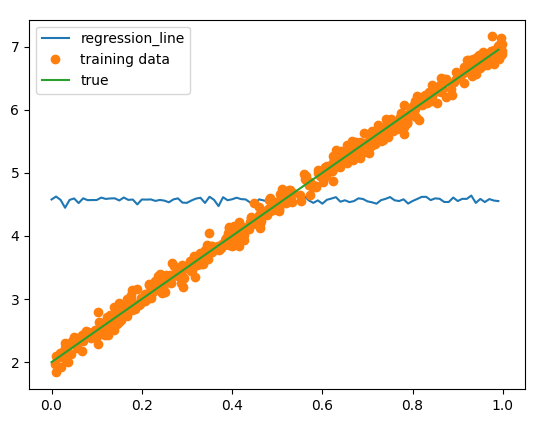# Learning linear function

Hi there!
I’m trying to use the pyro framework to learn the linear function `f(x)=5x+2` - for this I’ve worked through the Bayesian Regression Tutorial.

First I’ve designed some noisy data points of the function with

``````x_data = torch.rand((500, 1))
y_data = 5*x_data +2. + torch.normal(0, 0.1, size=(500, 1))
``````

Then I’ve build the Bayesian Regression Model. For a sanity check, I’ve set the priors of the weight and the bias to the true values and set a very low variance.

``````class BayesianRegression(PyroModule):
def __init__(self, in_features, out_features):
super().__init__()
self.linear = PyroModule[nn.Linear](in_features, out_features)
self.linear.weight = PyroSample(dist.Normal(5, 1).expand([out_features, in_features]).to_event(2))
self.linear.bias = PyroSample(dist.Normal(2, 1).expand([out_features]).to_event(1))

def forward(self, x, y=None):
sigma = pyro.sample("sigma", dist.Uniform(0., 1))
mean = self.linear(x).squeeze(-1)
with pyro.plate("data", x.shape):
obs = pyro.sample("obs", dist.Normal(mean, sigma), obs=y)
return mean
``````

Then I’ve ran the SVI for 1500 iterations:

``````model = BayesianRegression(1, 1)
guide = AutoDiagonalNormal(model)

adam = pyro.optim.Adam({"lr": 0.03})
svi = SVI(model, guide, adam, loss=Trace_ELBO())
pyro.clear_param_store()
for j in range(num_iterations):
# calculate the loss and take a gradient step
loss = svi.step(x_data, y_data)
if j % 100 == 0:
print("[iteration %04d] loss: %.4f" % (j + 1, loss / len(x_data)))
``````

Evaluating this model `x=torch.arange(0, 1, 0.01`) gives the following plot:After the training the mean of the weight is `0.0011` and the bias is `4.5674`.
I do not understand why these Means do not correspond to the correct values after the training, although the Priors already contain the desired value? Of course if I decrease the learning rate, the deviation from `5` and `2` is smaller after training, but this is obviously not the right way, because this only works because I already have the correct values in the prior.

Moreover if I e.g. choose other prios like

``````self.linear.weight = PyroSample(dist.Normal(0, 10).expand([out_features, in_features]).to_event(2))
self.linear.bias = PyroSample(dist.Normal(0, 10).expand([out_features]).to_event(1))
``````

no learning effect occurs,even after 10.000 training iterations, although the variance in the priors is pretty high.

What am I doing wrong?

I guess you need to call `svi.step(x_data, y_data.squeeze(-1))`?

1 Like

thank you so much! That’s it!